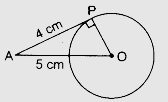# The length of a tangent from a point $A$ at distance 5 cm from the centre of the circle is 4 cm. Find the radius of the circle.

Given:

The length of a tangent from a point $A$ at distance 5 cm from the centre of the circle is 4 cm.

To do:

We have to find the radius of the circle.

Solution:

Let OP be the radius of a circle.$OAP$ is a right angled triangle.

Therefore,

$OA^2=AP^2+OP^2$

$5^{2}=4^{2}+\mathrm{OP}^{2}$25=16+\mathrm{OP}^{2}\mathrm{OP}^{2}=25-16\mathrm{OP}^{2}=9\mathrm{OP}=\sqrt{9}\mathrm{OP}=3 \mathrm{~cm}$The radius of the circle is$3 \mathrm{~cm}\$.

Updated on: 10-Oct-2022

37 Views